12.4.1 Step 2: Delete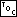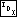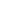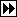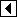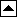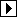We use left-looking deletion. At this point, p is the node to delete. After the deletion, x is the node that replaced p, or a null pointer if the node was deleted without replacement. The cases are distinguished in the usual way:

```469. <Step 2: Delete RTRB node 469> =
if (p->rtrb_link == NULL)   {
if (p->rtrb_rtag == RTRB_CHILD)
{         <Case 1 in RTRB deletion 470>       }
else       {         <Case 2 in RTRB deletion 471>       }
} else   {
enum rtrb_color t;
struct rtrb_node *r = p->rtrb_link;

if (r->rtrb_rtag == RTRB_THREAD)
{         <Case 3 in RTRB deletion 472>       }
else       {         <Case 4 in RTRB deletion 473>       }
}
```

This code is included in 468.

##### Case 1: p has a right child but no left child

If p, the node to be deleted, has a right child but no left child, then we replace it by its right child. This is the same as <Case 1 in RTAVL deletion 432>.

```470. <Case 1 in RTRB deletion 470> =
<Case 1 in RTAVL deletion; rtavl => rtrb 432>
```

This code is included in 469.

##### Case 2: p has a right thread and no left child

Similarly, case 2 is the same as <Case 2 in RTAVL deletion 433>, with the addition of an assignment to x.

```471. <Case 2 in RTRB deletion 471> =
<Case 2 in RTAVL deletion; rtavl => rtrb 433>
```

This code is included in 469.

##### Case 3: p's left child has a right thread

If p has a left child r, and r has a right thread, then we replace p by r and transfer p's former right link to r. Node r also receives p's balance factor.

```472. <Case 3 in RTRB deletion 472> =
r->rtrb_rtag = p->rtrb_rtag;
t = r->rtrb_color;
r->rtrb_color = p->rtrb_color;
p->rtrb_color = t;
pa[k - 1]->rtrb_link[da[k - 1]] = r;
da[k] = 0;
pa[k++] = r;
```

This code is included in 469.

##### Case 4: p's left child has a right child

The fourth case, where p has a left child that itself has a right child, uses the same algorithm as <Case 4 in RTAVL deletion 435>, except that instead of setting the balance factor of s, we swap the colors of t and s as in <Case 3 in RB deletion 224>.

```473. <Case 4 in RTRB deletion 473> =
struct rtrb_node *s;
int j = k++;

for (;;)   {
da[k] = 1;
pa[k++] = r;
if (s->rtrb_rtag == RTRB_THREAD)
break;

r = s;
}

da[j] = 0;
pa[j] = pa[j - 1]->rtrb_link[da[j - 1]] = s;

if (s->rtrb_link != NULL)
else   {